# 二、OpenCV 中的图像基础

• 图像基础的理论介绍
• 像素，颜色，通道，图像和色彩空间的概念
• OpenCV 中的坐标系
• 在 OpenCV 中访问和操作不同颜色空间中的像素（获取和设置）
• OpenCV 中的 BGR 顺序（而不是 RGB）

# 技术要求

• Python 和 OpenCV
• 特定于 Python 的 IDE
• NumPy 和 Matplotlib 包
• Jupyter 笔记本
• Git 客户端

# 图像处理中的主要问题

• 新的表示形式（例如，新的图像）
• 决策（例如，执行具体任务）
• 新结果（例如，图像的正确分类）
• 一些有用的信息提取（例如，对象检测）

• 含糊不清的图像，因为它们会受到透视的影响，这可能会导致图像的视觉外观发生变化。 例如，从不同角度观看的同一对象可能会产生不同的图像。
• 通常受许多因素影响的图像，例如照明，天气，反射和运动。
• 图像中的物体也可能被其他物体遮挡，从而难以检测或分类被遮挡的物体。 根据遮挡的级别，所需的任务（例如，将图像分类为一些预定义的类别）可能确实具有挑战性。

# 图像处理步骤

1. 获取要使用的图像。 此过程通常涉及一些函数，以便您可以从不同的来源（摄像机，视频流，磁盘，在线资源）读取图像。
2. 通过应用图像处理技术来处理图像以实现所需的功能（例如，检测图像中的猫）。
3. 显示处理步骤的结果（例如，在图像中绘制边框，然后将其保存到磁盘）。

• 低级流程
• 中级流程
• 高级流程

• 噪音消除
• 图像锐化
• 光照归一化
• 透视校正

• 人脸识别
• 情感识别
• 睡意和注意力分散
• 面部远程心率测量

# 图像创建

• x ∈ [0, h-1]，其中h是图像的高度
• y ∈ [0, w-1]，其中w是图像的宽度
• f(x, y) ∈ [0, L-1]，其中L = 256（对于 8 位图像）# 像素，颜色，通道，图像和色彩空间的概念

RGB 模型是一种加色模型，其中将原色(R, G, B)*混合在一起以再现各种颜色。 如前所述，在 RGB 模型中，原色是红色，绿色和蓝色。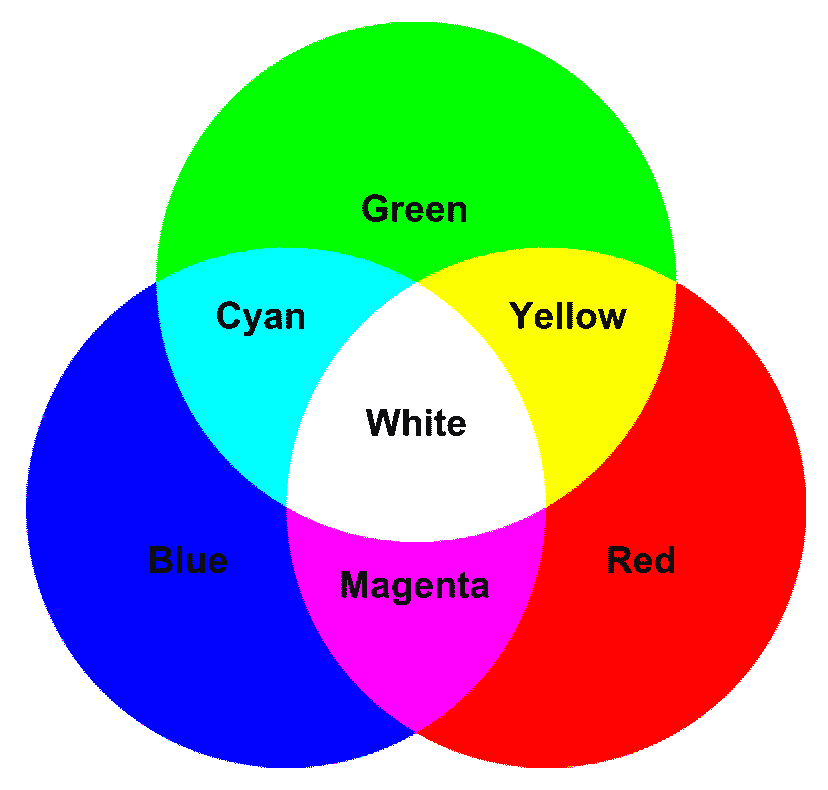• 将红色添加到绿色将获得黄色
• 将红色添加到蓝色会产生洋红色
• 将绿色添加到蓝色会生成青色
• 将所有三种原色相加会产生白色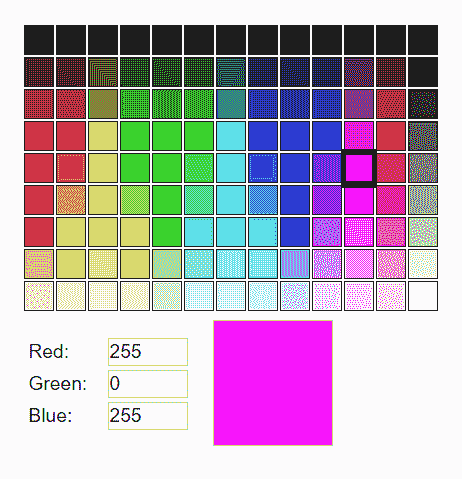PPI = 图像的宽度（像素）/ 宽度（英寸）
PPI = 图像的高度（像素）/ 高度（英寸）


# 文件扩展名

• Windows 位图*.bmp*.dib
• JPEG 文件*.jpeg*.jpg*.jpe
• JPEG 2000 文件*.jp2
• 便携式网络图形*.png
• 便携式图像格式*.pbm*.pgm*.ppm
• TI​​FF 文件*.tiff*.tif

JPEG 2000 是图像压缩标准和编码系统，它使用基于小波的压缩技术来提供高级别的可伸缩性和可访问性。 以此方式，JPEG 2000 压缩的图像比常规 JPEG 少。

# OpenCV 中的坐标系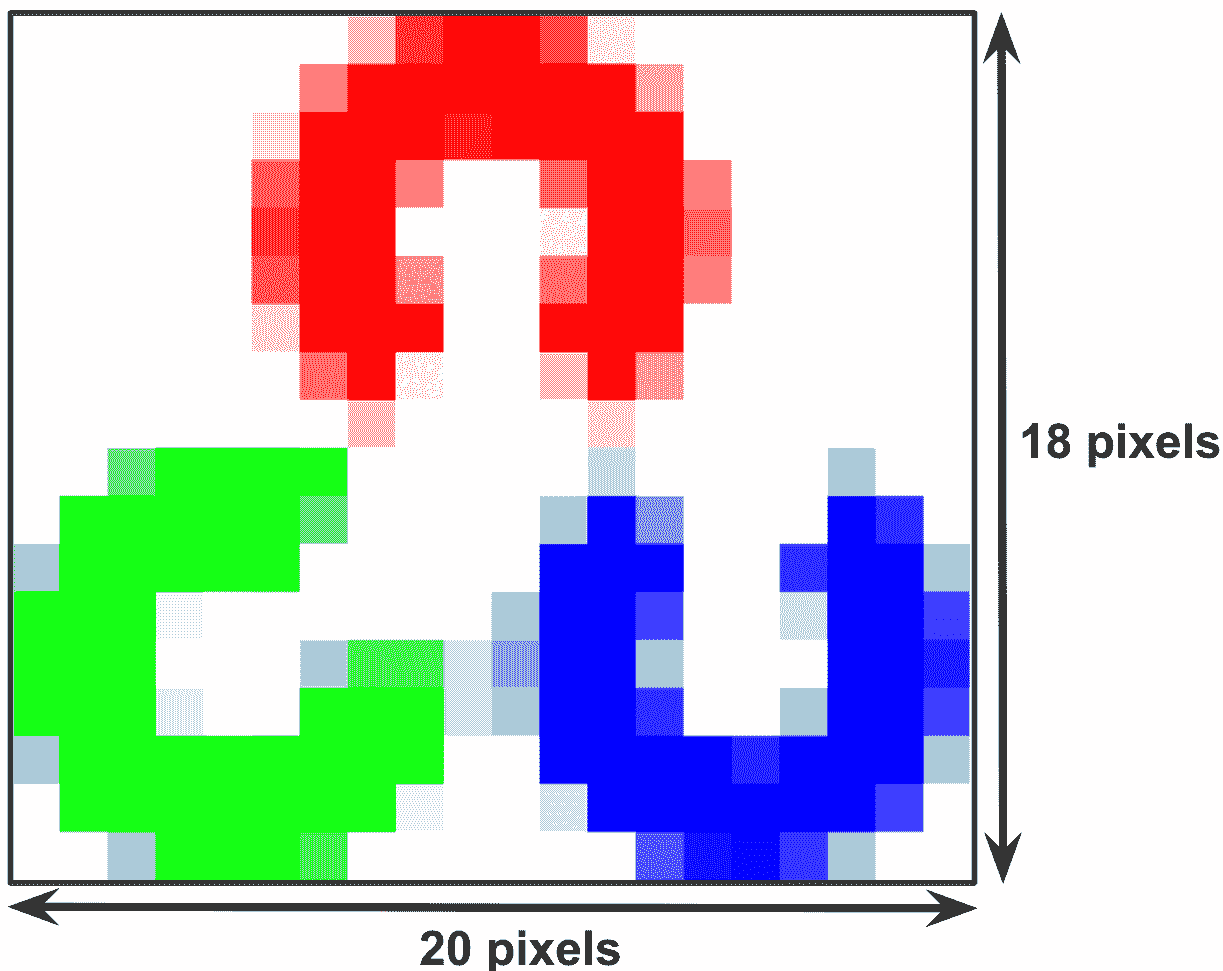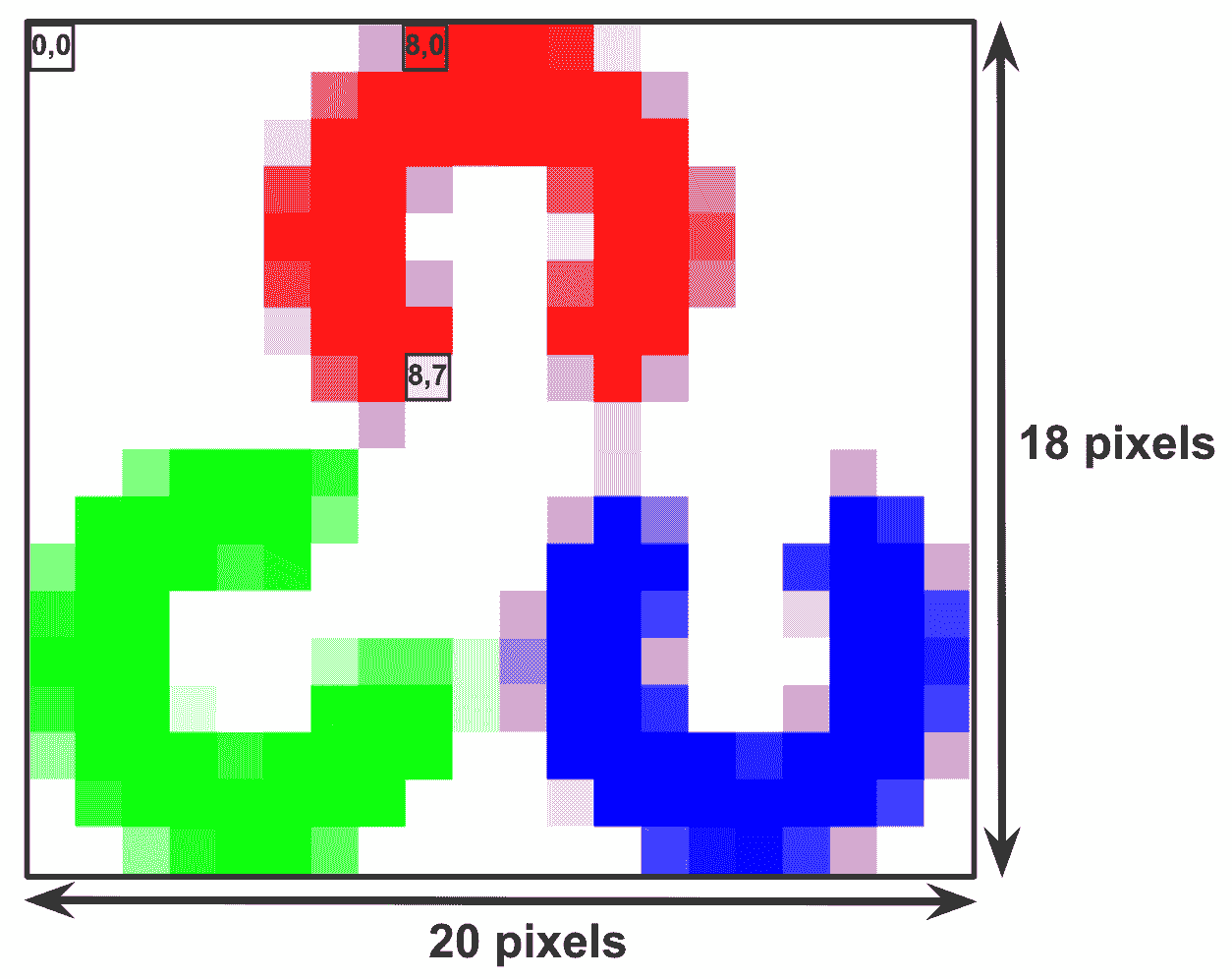# 在 OpenCV 中访问和操纵 BGR 图像的像素

# The function cv2.imread() is used to read an image from the the working directory
# Alternatively, you should provide a full path of the image:
# Load OpenCV logo image (in this case from the working directoy):


# To get the dimensions of the image use img.shape
# img.shape returns a tuple of number of rows, columns and channels (if a colour image)
# If image is grayscale, img.shape returns a tuple of number of rows and columns.
# So,it can be used to check if loaded image is grayscale or color image.
# Get the shape of the image:
dimensions = img.shape


# Total number of elements is obtained by img.size:
total_number_of_elements= img.size


# Image datatype is obtained by img.dtype.
# img.dtype is very important because a large number of errors is caused by invalid datatype.
# Get the image datatype:
image_dtype = img.dtype


# The function cv2.imshow() is used to display an image in a window
# The first argument of this function is the window name
# The second argument of this function is the image to be shown.
# Each created window should have different window names.
# Show original image:
cv2.imshow("original image", img)


# The function cv2.waitKey(), which is a keyboard binding function, waits for any keyboard event.
# This function waits the value indicated by the argument (in milliseconds).
# If any keyboard event is produced in this period of time, the program continues its execution
# If the value of the argument is 0, the program waits indefinitely until a keyboard event is produced:
cv2.waitKey(0)


# A pixel value can be accessed by row and column coordinates.
# In case of BGR image, it returns an array of (Blue, Green, Red) values.
# Get the value of the pixel (x=40, y=6):
(b, g, r) = img[6, 40]


# We can only  access one channel at a time.
# In this case, we will use row, column and the index of the desired channel for indexing.
# Get only blue value of the pixel (x=40, y=6):
b = img[6, 40, 0]


# The pixel values can be also modified in the same way - (b, g, r) format:
img[6, 40] = (0, 0, 255)


# In this case, we get the top left corner of the image:
top_left_corner = img[0:50, 0:50]


top_left_corner变量是另一张图像（小于img），但是我们可以用相同的方式来播放它。

# 在 OpenCV 中访问和操作灰度图像的像素

# The function cv2.imshow() is used to display an image in a window
# The first argument of this function is the window name
# The second argument of this function is the image to be shown.
# In this case, the second argument is needed because we want to load the image in grayscale.
# Second argument is a flag specifying the way the image should be read.


# To get the dimensions of the image use img.shape
# If color image, img.shape returns returns a tuple of number of rows, columns and channels
# If grayscale, returns a tuple of number of rows and columns.
# So, it can be used to check if the loaded image is grayscale or color image.
# Get the shape of the image (in this case only two components!):
dimensions = gray_img.shape


img.shape将以元组的形式返回图像的尺寸，例如(99, 82)

# You can access a pixel value by row and column coordinates.
# For BGR image, it returns an array of (Blue, Green, Red) values.
# Get the value of the pixel (x=40, y=6):
i = gray_img[6, 40]


# You can modify the pixel values of the image in the same way.
# Set the pixel to black:
gray_img[6, 40] = 0


# OpenCV 中的 BGR 顺序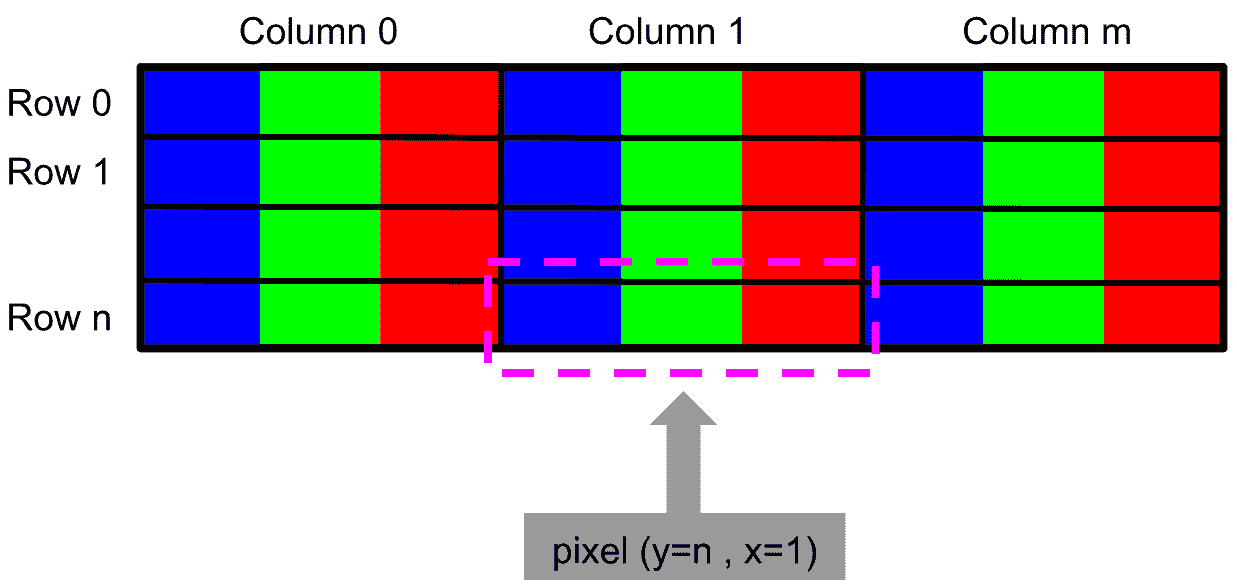OpenCV 的最初开发人员选择了 BGR 色彩格式（而不是 RGB 格式），因为当时 BGR 色彩格式在软件提供商和相机制造商中非常受欢迎。 例如，在 Windows 中，使用 COLORREF 指定颜色值时，他们使用 BGR 格式0x00bbggrr。 总而言之，选择 BGR 是出于历史原因。

# Load image using cv2.imread:


# Split the loaded image into its three channels (b, g, r):
b, g, r = cv2.split(img_OpenCV)


# Merge again the three channels but in the RGB format:
img_matplotlib = cv2.merge([r, g, b])


# Show both images (img_OpenCV and img_matplotlib) using matplotlib
# This will show the image in wrong color:
plt.subplot(121)
plt.imshow(img_OpenCV)
# This will show the image in true color:
plt.subplot(122)
plt.imshow(img_matplotlib)
plt.show()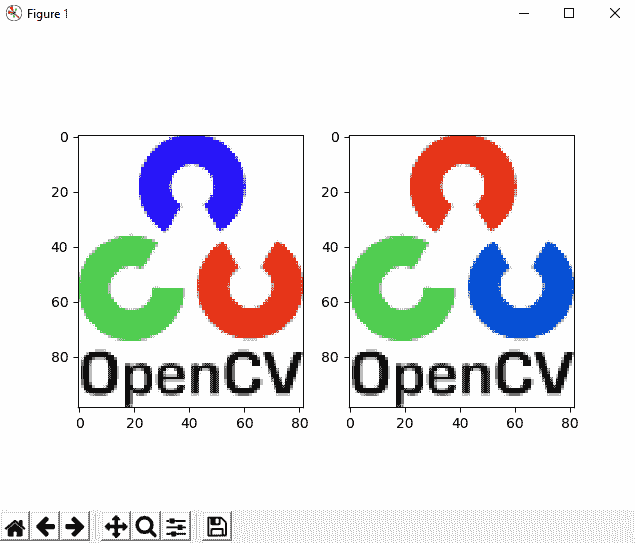# Show both images (img_OpenCV and img_matplotlib) using cv2.imshow()
# This will show the image in true color:
cv2.imshow('bgr image', img_OpenCV)
# This will show the image in wrong color:
cv2.imshow('rgb image', img_matplotlib)
cv2.waitKey(0)
cv2.destroyAllWindows()# To stack horizontally (img_OpenCV to the left of img_matplotlib):
img_concats = np.concatenate((img_OpenCV, img_matplotlib), axis=1)
# Now, we show the concatenated image:
cv2.imshow('bgr image and rgb image', img_concats)
cv2.waitKey(0)
cv2.destroyAllWindows()# Using numpy capabilities to get the channels and to build the RGB image
# Get the three channels (instead of using cv2.split):
B = img_OpenCV[:, :, 0]
G = img_OpenCV[:, :, 1]
R = img_OpenCV[:, :, 2]


# Transform the image BGR to RGB using Numpy capabilities:
img_matplotlib = img_OpenCV[:, :, ::-1]


• Getting-And-Setting-BGR.ipynb
• Getting-And-Setting-GrayScale.ipynb

\$ jupyter notebook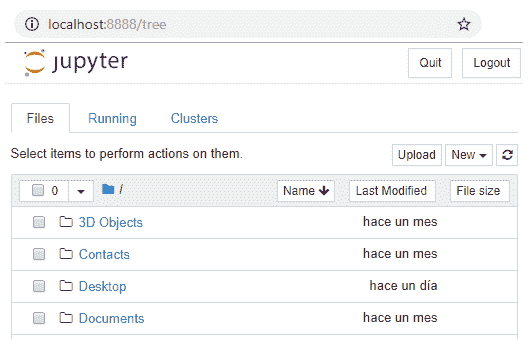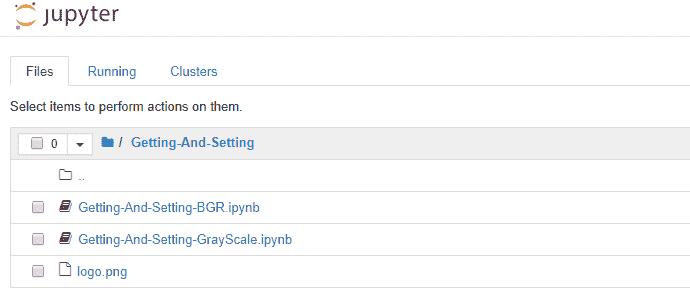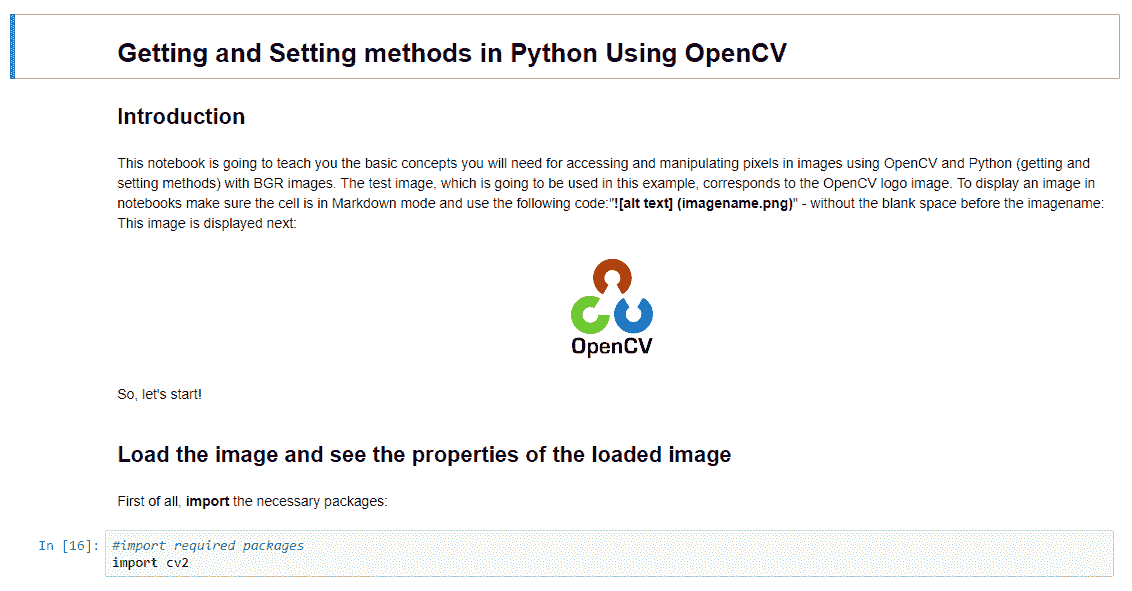# 总结

• 访问图像属性
• 一些 OpenCV 函数，例如cv2.imread()cv2.split()cv2.merge()cv2.imshow()cv2.waitKey()cv2.destroyAllWindows()
• 如何在 BGR 和灰度图像中获取和设置图像像素

# 问题

1. 主要的图像处理步骤是什么？
2. 三种处理级别是什么？
3. 灰度图像和黑白图像有什么区别？
4. 什么是像素？
5. 什么是图像分辨率？
6. 您使用哪些 OpenCV 函数执行以下操作？
• 加载（读取）图像
• 显示图像
• 等待按键
• 拆分通道
• 合并通道
7. 您使用什么命令来运行 Jupyter 笔记本？
8. 以下三元组会得到什么颜色？
• B = 0G = 255R = 255
• B = 255G = 255R = 0
• B = 255G = 0R = 255
• B = 255G = 255R = 255
9. 假设您已在img中加载了图像。 如何检查img是彩色还是灰度？

# 进一步阅读

• 有关 Git 的更多信息，请看这本书：

《精通 Git》，作者 JakubNarębski

• 有关 Jupyter 笔记本的更多信息：

《Jupyter 笔记本：第一部分》，作者 Dan Toomey